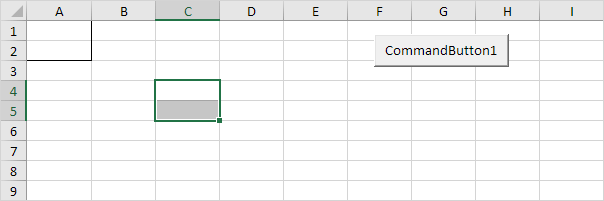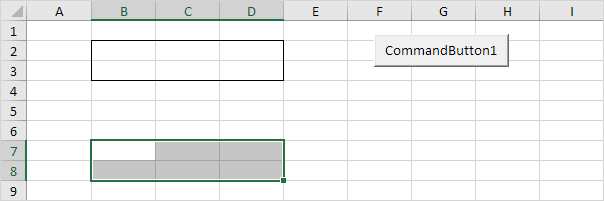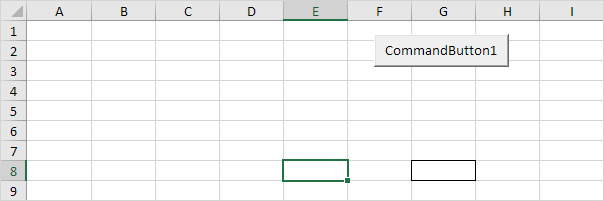# Offset

The Offset property in Excel VBA takes the range which is a particular number of rows and columns away from a certain range.

Place a command button on your worksheet and add the following code lines:

1. The Offset property below returns a range which is 3 rows below and 2 columns to the right of a range.

Range("A1:A2").Offset(3, 2).SelectExplanation: the Select method selects this range. The Offset property always takes the top left cell of a range as the starting point. Border for illustration only.

2. The Offset property below returns a range which is 1 row above and 4 columns to the left of a range.

Range("F5:H5").Offset(-1, -4).Select3. You can also offset by a number of rows only. For example, return a range which is 5 rows below a range.

Range("B2:D3").Offset(5).Select4. Finally, you can also offset by a number of columns only. For example, return a cell which is 2 columns to the left of a cell.

Range("G8").Offset(0, -2).SelectFor a practical example of the offset property, see our example program Create a Pattern.

Go to Next Chapter: Variables